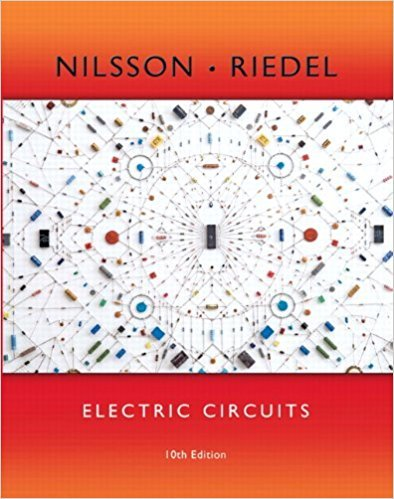×
Get Full Access to Electric Circuits - 10 Edition - Chapter 4 - Problem 4.7
Get Full Access to Electric Circuits - 10 Edition - Chapter 4 - Problem 4.7

×

# Solved: a) Find the power developed by the 40 mA current source in the circuit in FigISBN: 9780133760033 390

## Solution for problem 4.7 Chapter 4

Electric Circuits | 10th Edition

• Textbook Solutions
• 2901 Step-by-step solutions solved by professors and subject experts
• Get 24/7 help from StudySoup virtual teaching assistantsElectric Circuits | 10th Edition

4 5 1 404 Reviews
29
2
Problem 4.7

a) Find the power developed by the 40 mA current source in the circuit in Fig. P4.6. b) Find the power developed by the 24 V voltage source in the circuit in Fig. P4.6. c) Verify that the total power developed equals the total power dissipated.

Step-by-Step Solution:
Step 1 of 3

Emily Ballentine eballen@g.clemson.edu ECON 2110­41 Notes 9/21/17 – 9/28/17 1 8.5: Temperature  Actual Temperature o Use an equation when solving a problem concerning an actual temperature.  Change in Temperature o Use a conversion factor when solving a problem concerning ΔTemp.  Material Property o Use a conversion factor when solving a problem concerning material properties.  Temperature Equations T [ ] T ℃[ ]2 o 5 o T K =T ℃ +273 T ° ]=T ℉ ]+460 o  Temperature Conversion Factors 1℉ o 1° R 1℃ o 1K 1℃ o 1.8℉ 8.9-8.10: Power and Efficie

Step 2 of 3

Step 3 of 3

##### ISBN: 9780133760033

Unlock Textbook Solution